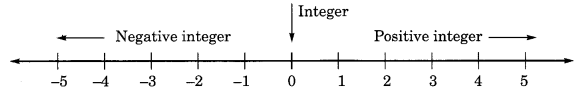On this page, you will find Integers Class 6 Notes Maths Chapter 6 Pdf free download. CBSE NCERT Class 6 Maths Notes Chapter 6 Integers will seemingly help them to revise the important concepts in less time.

## CBSE Class 6 Maths Chapter 6 Notes Integers

### Integers Class 6 Notes Conceptual Facts

1. On a number line, the numbers left to 0 are called negative numbers.2. Natural numbers are 1, 2, 3, 4, ……….

3. Whole numbers are 0, 1, 2, 3, 4, ……….

4. Negative numbers are , – 4, – 3, – 2, – 1.

5. Integers are , – 4, – 3, – 2, – 1, 0, 1, 2, 3, 4,………..

6. 0 is simply called an integer. It has no sign.7. Positive and negative integers are used in daily life for profit and loss, rise and fall, above and below, etc.

8. Negative of a negative integer = positive integer, i.e., – (- x) – x

9. Every positive number is larger than every negative number.

10. Farther a number from zero on the right, larger is its value.

11. Farther a number from zero on the left, smaller is its value.

12. Absolute value is a numerical value of a number which is represented by ||.

13. The absolute value of+ 5= | + 5| = 5

• Absolute value of — 5 = | — 5 | =5
• Absolute value of 0 is 0 i.e., | 0 | =0